# 十大经典排序算法最强总结（含JAVA代码实现）

0、排序算法说明

0.1 排序的定义

0.2 术语说明

• 稳定：如果a原本在b前面，而a=b，排序之后a仍然在b的前面；

• 不稳定：如果a原本在b的前面，而a=b，排序之后a可能会出现在b的后面；

• 内排序：所有排序操作都在内存中完成；

• 外排序：由于数据太大，因此把数据放在磁盘中，而排序通过磁盘和内存的数据传输才能进行；

• 时间复杂度： 一个算法执行所耗费的时间。

• 空间复杂度：运行完一个程序所需内存的大小。

• https://www.cnblogs.com/yongh/p/9637260.html

0.3 算法总结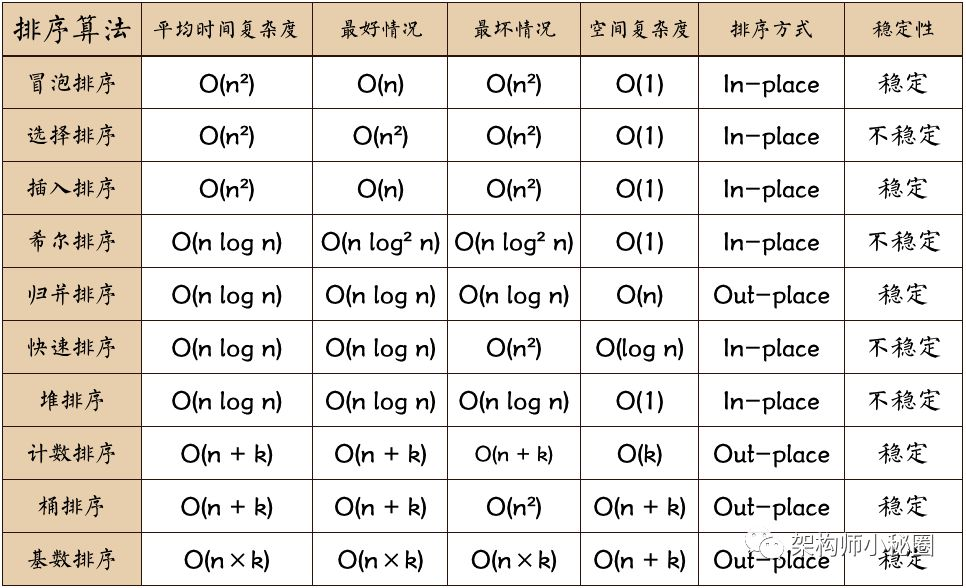• n: 数据规模

• k: “桶”的个数

• In-place: 占用常数内存，不占用额外内存

• Out-place: 占用额外内存

0.5 算法分类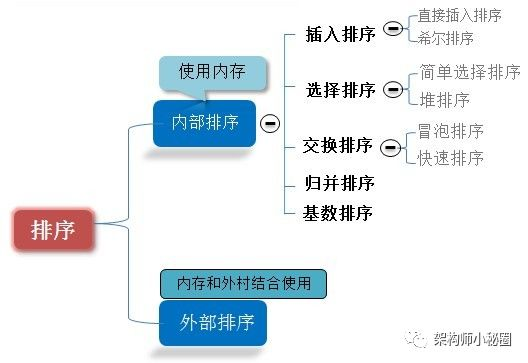0.6 比较和非比较的区别

1、冒泡排序（Bubble Sort）

1.1 算法描述

• 比较相邻的元素。如果第一个比第二个大，就交换它们两个；

• 对每一对相邻元素作同样的工作，从开始第一对到结尾的最后一对，这样在最后的元素应该会是最大的数；

• 针对所有的元素重复以上的步骤，除了最后一个；

• 重复步骤1~3，直到排序完成。

1.2 动图演示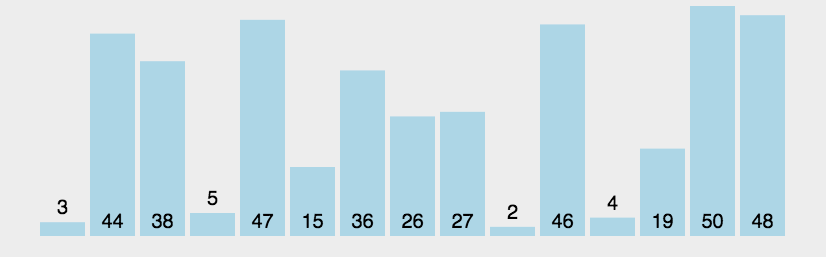1.3 代码实现

/**
* 冒泡排序
*
* @param array
* @return
*/
public static int[] bubbleSort(int[] array) {
if (array.length == 0)
return array;
for (int i = 0; i < array.length; i++)
for (int j = 0; j < array.length - 1 - i; j++)
if (array[j + 1] < array[j]) {
int temp = array[j + 1];
array[j + 1] = array[j];
array[j] = temp;
}
return array;
}

1.4 算法分析

2、选择排序（Selection Sort）

2.1 算法描述

n个记录的直接选择排序可经过n-1趟直接选择排序得到有序结果。具体算法描述如下：

• 初始状态：无序区为R[1..n]，有序区为空；

• 第i趟排序(i=1,2,3…n-1)开始时，当前有序区和无序区分别为R[1..i-1]和R(i..n）。该趟排序从当前无序区中-选出关键字最小的记录 R[k]，将它与无序区的第1个记录R交换，使R[1..i]和R[i+1..n)分别变为记录个数增加1个的新有序区和记录个数减少1个的新无序区；

• n-1趟结束，数组有序化了。

2.2 动图演示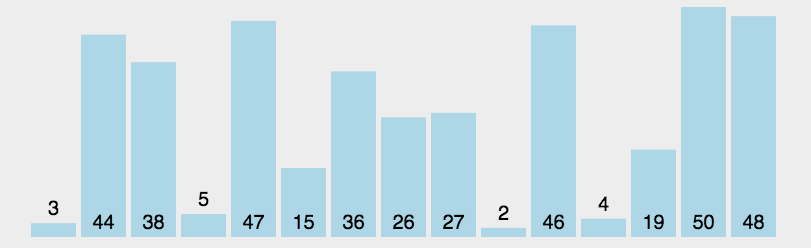2.3 代码实现

  /**
* 选择排序
* @param array
* @return
*/
public static int[] selectionSort(int[] array) {
if (array.length == 0)
return array;
for (int i = 0; i < array.length; i++) {
int minIndex = i;
for (int j = i; j < array.length; j++) {
if (array[j] < array[minIndex]) //找到最小的数
minIndex = j; //将最小数的索引保存
}
//找到最小的下标与当前i进行交换
int temp = array[minIndex];
array[minIndex] = array[i];
array[i] = temp;
}
return array;
}

2.4 算法分析

3、插入排序（Insertion Sort）

3.1 算法描述

• 从第一个元素开始，该元素可以认为已经被排序；

• 取出下一个元素，在已经排序的元素序列中从后向前扫描；

• 如果该元素（已排序）大于新元素，将该元素移到下一位置；

• 重复步骤3，直到找到已排序的元素小于或者等于新元素的位置；

• 将新元素插入到该位置后；

• 重复步骤2~5。

3.2 动图演示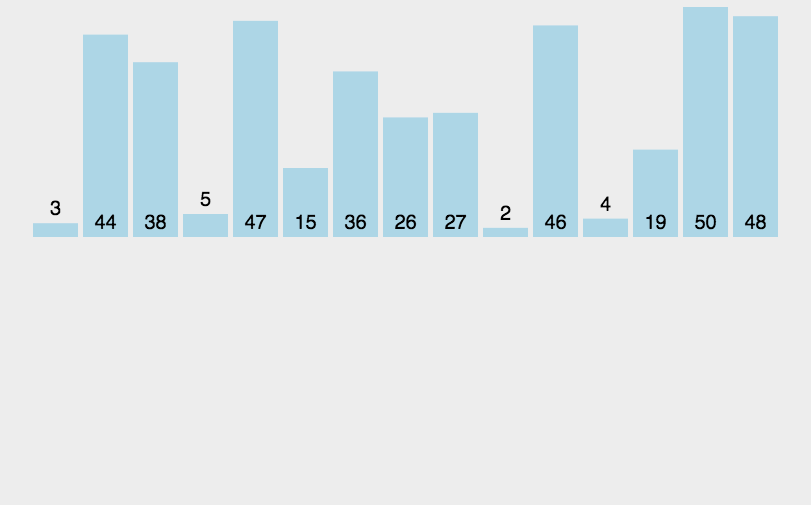3.3 代码实现

	/**
* 插入排序 思路：把数组拆分成两个数组：已排序与未排序的数组，前面是已排序的数组 后面是未排序的数组
*  解决问题：
* 1.当前要插入的值
* 2.比较的位置下标
* */

public static int[] insertSort(int[] array) {
if (array.length == 0) {
return array;
}
int curraIndex;// 当前下标
int premDate;// 要插入的值
for (int index = 0; index < array.length - 1; index++) {
curraIndex = index;// 把已经排序的数组最大的下标给当前下标
premDate = array[index + 1];// 没有排序的数组第一个数值即将要排序的数值
while (curraIndex >= 0 && premDate < array[curraIndex]) {// 如果当前值premDate比已经的排序数组的值小则继续往下比较直到小于为止
array[curraIndex + 1] = array[curraIndex];// 数组值往后平移一位
curraIndex--;// 减一是为了往下个比较
}
array[curraIndex + 1] = premDate;// 把当前要插入的数值插入到第一个比它小的数值前面
}
print(array);
return array;
}
/**
* 插入排序
* @param array
* @return
*/
public static int[] insertionSort(int[] array) {
if(array.length == 0){
return array;
}
int current;
for(int i = 0; i < array.length-1;i++){
current = array[i+1];//当前要插入的数据
int preIndex = i;//前面逐渐要比较的值的下标
while(preIndex >= 0 && current < array[preIndex]){
array[preIndex+1] =  array[preIndex];
preIndex--;//逐渐减小下标进行比较
}
array[preIndex+1] = current; //把当前数值插入到比当前值小的前面
}

return array;
}

3.4 算法分析

4、希尔排序（Shell Sort）

4.1 算法描述

• 选择一个增量序列t1，t2，…，tk，其中ti>tj，tk=1；

• 按增量序列个数k，对序列进行k 趟排序；

• 每趟排序，根据对应的增量ti，将待排序列分割成若干长度为m 的子序列，分别对各子表进行直接插入排序。仅增量因子为1 时，整个序列作为一个表来处理，表长度即为整个序列的长度。

4.2 过程演示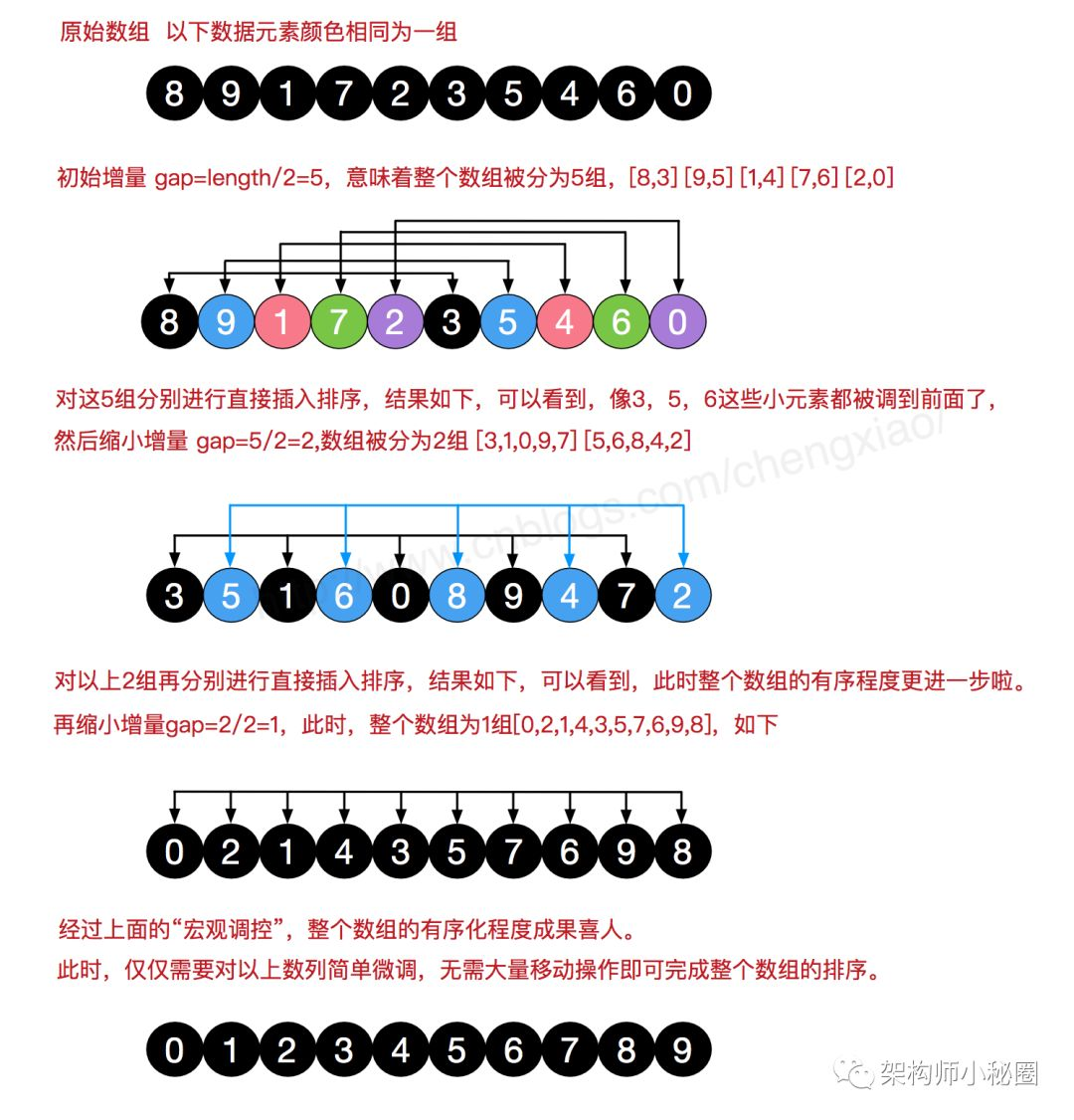4.3 代码实现

 /**
* 希尔排序
*
* @param array
* @return
*/
public static int[] ShellSort(int[] array) {
int len = array.length;
int temp, gap = len / 2;
while (gap > 0) {
for (int i = gap; i < len; i++) {
temp = array[i];
int preIndex = i - gap;
while (preIndex >= 0 && array[preIndex] > temp) {
array[preIndex + gap] = array[preIndex];
preIndex -= gap;
}
array[preIndex + gap] = temp;
}
gap /= 2;
}
return array;
}
 /**
* 希尔排序
*
* @param array
* @return
*/
public static int[] shellSort(int[] array) {
if(array.length == 0) return array;
int gep = array.length /2;
int premDate = 0;
while(gep > 0){
for(int i = gep; i < array.length;i++ ){
int curraIndex = i-gep;//当前下标
premDate = array[i]; //要插入的值
while(curraIndex >= 0 && premDate < array[curraIndex]){// 如果当前值premDate比已经的排序数组的值小则继续往下比较直到小于为止
array[curraIndex+gep] = array[curraIndex];//数组值往后平移gep位
curraIndex -= gep;
}
array[curraIndex+gep] = premDate;
}
gep /=2;
}
return array;
}


4.4 算法分析

5、归并排序（Merge Sort）

5.1 算法描述

• 把长度为n的输入序列分成两个长度为n/2的子序列；

• 对这两个子序列分别采用归并排序；

• 将两个排序好的子序列合并成一个最终的排序序列。

5.2 动图演示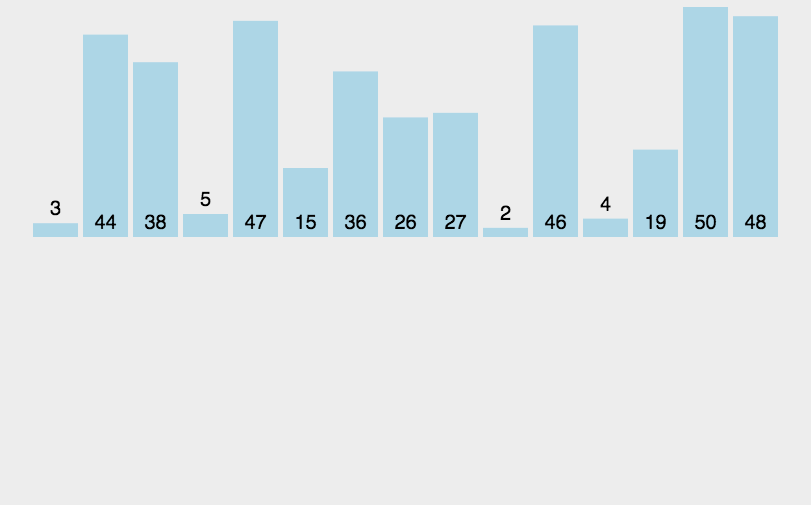5.3 代码实现

/**
* 归并排序
*
* @param array
* @return
*/
public static int[] MergeSort(int[] array) {
if (array.length < 2) return array;
int mid = array.length / 2;
int[] left = Arrays.copyOfRange(array, 0, mid);
int[] right = Arrays.copyOfRange(array, mid, array.length);
return merge(MergeSort(left), MergeSort(right));
}
/**
* 归并排序——将两段排序好的数组结合成一个排序数组
*
* @param left
* @param right
* @return
*/
public static int[] merge(int[] left, int[] right) {
int[] result = new int[left.length + right.length];
for (int index = 0, i = 0, j = 0; index < result.length; index++) {
if (i >= left.length)
result[index] = right[j++];
else if (j >= right.length)
result[index] = left[i++];
else if (left[i] > right[j])
result[index] = right[j++];
else
result[index] = left[i++];
}
return result;
}


5. 4 算法分析

6、快速排序（Quick Sort）

6.1 算法描述

• 从数列中挑出一个元素，称为 “基准”（pivot）；

• 重新排序数列，所有元素比基准值小的摆放在基准前面，所有元素比基准值大的摆在基准的后面（相同的数可以到任一边）。在这个分区退出之后，该基准就处于数列的中间位置。这个称为分区（partition）操作；

• 递归地（recursive）把小于基准值元素的子数列和大于基准值元素的子数列排序。

6.2 动图演示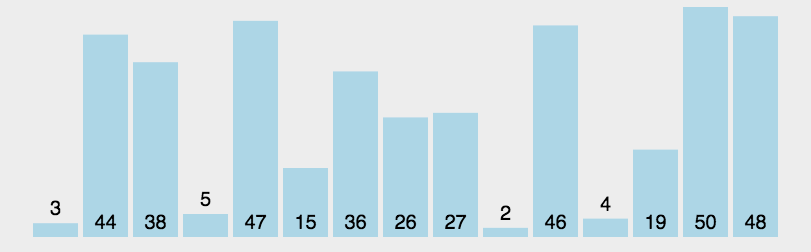6.3 代码实现

/**
* 快速排序方法
* @param array
* @param start
* @param end
* @return
*/
public static int[] QuickSort(int[] array, int start, int end) {
if (array.length < 1 || start < 0 || end >= array.length || start > end) return null;
int smallIndex = partition(array, start, end);
if (smallIndex > start)
QuickSort(array, start, smallIndex - 1);
if (smallIndex < end)
QuickSort(array, smallIndex + 1, end);
return array;
}
/**
* 快速排序算法——partition
* @param array
* @param start
* @param end
* @return
*/
public static int partition(int[] array, int start, int end) {
int pivot = (int) (start + Math.random() * (end - start + 1));
int smallIndex = start - 1;
swap(array, pivot, end);
for (int i = start; i <= end; i++)
if (array[i] <= array[end]) {
smallIndex++;
if (i > smallIndex)
swap(array, i, smallIndex);
}
return smallIndex;
}
/**
* 交换数组内两个元素
* @param array
* @param i
* @param j
*/
public static void swap(int[] array, int i, int j) {
int temp = array[i];
array[i] = array[j];
array[j] = temp;
}

/**
* 获取快排的中间位置
* */
public static int getmindIndex(int[] array, int low, int high) {
int temp = array[low];// 把第一个数据作为比较的数据
while (low < high) {
while (low < high && array[high] > temp) {// 从右边往左边找到比temp小的数
high--;
}
// 表示找到了小于temp的元素
if (low < high)
swap(array, low++, high);// 交换后low执行+1操作
while (low < high && array[low] < temp) {// 从左边往右边找到比temp大的数据
low++;
}
// 表示找到了大于temp的元素
if (low < high)
// 交换后high执行-1操作
swap(array, low, high--);
print(array);
System.out.println("  ");
System.out.println("当前low="+low+",当前high="+high);
}
System.out.println("比完一真趟。。。。。");
return high; // 返回中轴的位置
}

/**
* 快速排序
* */
public static void quickSort(int[] array, int low, int high) {
if (low >= high) {
return;
}
// if(low <high){
int middle = getmindIndex(array, low, high);
System.out.println("返回的中间位置："+middle);
quickSort(array, low, middle - 1);
quickSort(array, middle + 1, high);
}

/**
* 交换函数，实现数组中两个数的交换操作
*
* @param array
*            待操作数组
* @param i
*            交换数组的第一个下标
* @param j
*            交换数组的第二个下标
*/
public static void swap(int[] array, int i, int j) {
int temp = array[i];
array[i] = array[j];
array[j] = temp;
}

public static void print(int[] array) {
for (int index = 0; index < array.length; index++) {
System.out.print(array[index] + "  ");
}
}

public static void main(String[] args) {
// TODO Auto-generated method stub
/*int[] array = new int[] { 30, 45, 78, 64, 52, 11, 64, 55, 99, 18, 15,
89, 100, 20, 32 };*/

int[] array = new int[] { 30, 45, 78, 100, 52, 11, 64, 55 };
// selectionSort(array);
// insertSort(array);
quickSort(array, 0, array.length - 1);
print(array);
System.out.println("执行完了。。。");
}


11  30  78  100  52  45  64  55

11  30  78  100  52  45  64  55

11  30  55  78  52  45  64  100

11  30  55  64  52  45  78  100

11  30  45  55  52  64  78  100

11  30  45  52  55  64  78  100

11  30  45  52  55  64  78  100

11  30  45  52  55  64  78  100  执行完了。。。


6.4 算法分析

7、堆排序（Heap Sort）

7.1 算法描述

• 将初始待排序关键字序列(R1,R2….Rn)构建成大顶堆，此堆为初始的无序区；

• 将堆顶元素R与最后一个元素R[n]交换，此时得到新的无序区(R1,R2,……Rn-1)和新的有序区(Rn),且满足R[1,2…n-1]<=R[n]；

• 由于交换后新的堆顶R可能违反堆的性质，因此需要对当前无序区(R1,R2,……Rn-1)调整为新堆，然后再次将R与无序区最后一个元素交换，得到新的无序区(R1,R2….Rn-2)和新的有序区(Rn-1,Rn)。不断重复此过程直到有序区的元素个数为n-1，则整个排序过程完成。

7.2 动图演示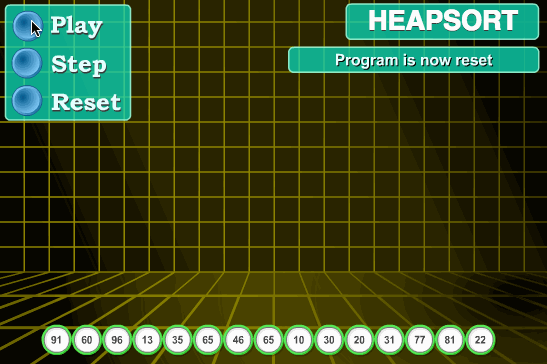7.3 代码实现

//声明全局变量，用于记录数组array的长度；
static int len;
/**
* 堆排序算法
*
* @param array
* @return
*/
public static int[] HeapSort(int[] array) {
len = array.length;
if (len < 1) return array;
//1.构建一个最大堆
buildMaxHeap(array);
//2.循环将堆首位（最大值）与末位交换，然后在重新调整最大堆
while (len > 0) {
swap(array, 0, len - 1);
len--;
}
return array;
}
/**
* 建立最大堆
*
* @param array
*/
public static void buildMaxHeap(int[] array) {
//从最后一个非叶子节点开始向上构造最大堆
for (int i = (len - 1) / 2; i >= 0; i--) {
}
}
/**
* 调整使之成为最大堆
*
* @param array
* @param i
*/
public static void adjustHeap(int[] array, int i) {
int maxIndex = i;
//如果有左子树，且左子树大于父节点，则将最大指针指向左子树
if (i * 2 < len && array[i * 2] > array[maxIndex])
maxIndex = i * 2;
//如果有右子树，且右子树大于父节点，则将最大指针指向右子树
if (i * 2 + 1 < len && array[i * 2 + 1] > array[maxIndex])
maxIndex = i * 2 + 1;
//如果父节点不是最大值，则将父节点与最大值交换，并且递归调整与父节点交换的位置。
if (maxIndex != i) {
swap(array, maxIndex, i);
}
}

7.4 算法分析

8、计数排序（Counting Sort）

8.1 算法描述

• 找出待排序的数组中最大和最小的元素；

• 统计数组中每个值为i的元素出现的次数，存入数组C的第i项；

• 对所有的计数累加（从C中的第一个元素开始，每一项和前一项相加）；

• 反向填充目标数组：将每个元素i放在新数组的第C(i)项，每放一个元素就将C(i)减去1。

8.2 动图演示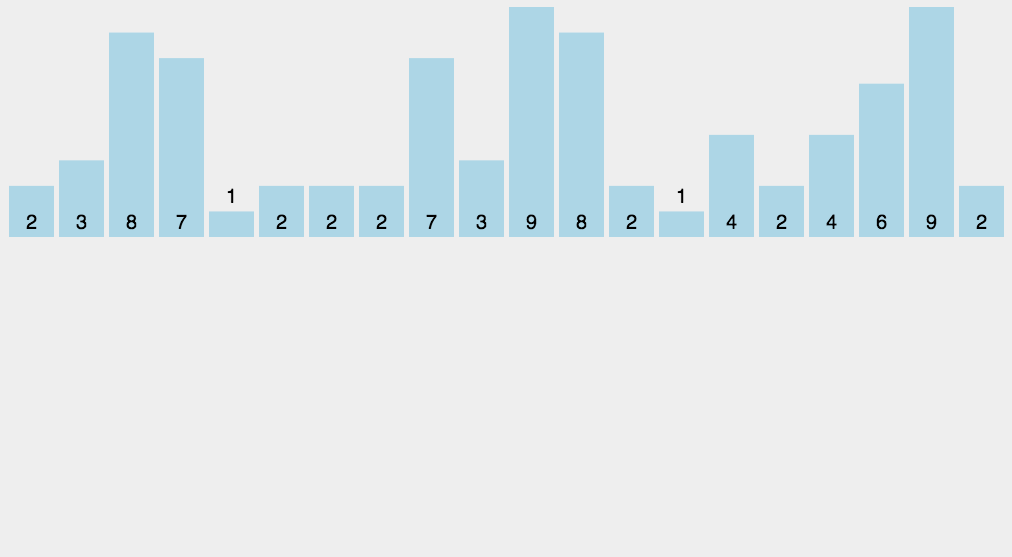8.3 代码实现

/**
* 计数排序
*
* @param array
* @return
*/
public static int[] CountingSort(int[] array) {
if (array.length == 0) return array;
int bias, min = array, max = array;
for (int i = 1; i < array.length; i++) {
if (array[i] > max)
max = array[i];
if (array[i] < min)
min = array[i];
}
bias = 0 - min;
int[] bucket = new int[max - min + 1];
Arrays.fill(bucket, 0);
for (int i = 0; i < array.length; i++) {
bucket[array[i] + bias]++;
}
int index = 0, i = 0;
while (index < array.length) {
if (bucket[i] != 0) {
array[index] = i - bias;
bucket[i]--;
index++;
} else
i++;
}
return array;
}

8.4 算法分析

9、桶排序（Bucket Sort）

9.1 算法描述

• 人为设置一个BucketSize，作为每个桶所能放置多少个不同数值（例如当BucketSize==5时，该桶可以存放｛1,2,3,4,5｝这几种数字，但是容量不限，即可以存放100个3）；

• 遍历输入数据，并且把数据一个一个放到对应的桶里去；

• 对每个不是空的桶进行排序，可以使用其它排序方法，也可以递归使用桶排序；

• 从不是空的桶里把排好序的数据拼接起来。

9.2 图片演示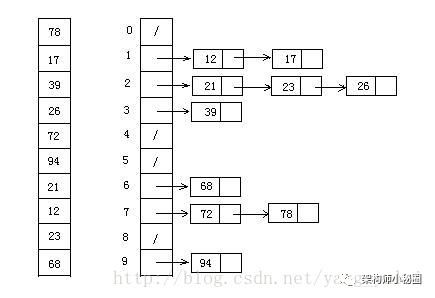9.3 代码实现

/**
* 桶排序
*
* @param array
* @param bucketSize
* @return
*/
public static ArrayList<Integer> BucketSort(ArrayList<Integer> array, int bucketSize) {
if (array == null || array.size() < 2)
return array;
int max = array.get(0), min = array.get(0);
// 找到最大值最小值
for (int i = 0; i < array.size(); i++) {
if (array.get(i) > max)
max = array.get(i);
if (array.get(i) < min)
min = array.get(i);
}
int bucketCount = (max - min) / bucketSize + 1;
ArrayList<ArrayList<Integer>> bucketArr = new ArrayList<>(bucketCount);
ArrayList<Integer> resultArr = new ArrayList<>();
for (int i = 0; i < bucketCount; i++) {
}
for (int i = 0; i < array.size(); i++) {
bucketArr.get((array.get(i) - min) / bucketSize).add(array.get(i));
}
for (int i = 0; i < bucketCount; i++) {
if (bucketCount == 1)
bucketSize--;
ArrayList<Integer> temp = BucketSort(bucketArr.get(i), bucketSize);
for (int j = 0; j < temp.size(); j++)
}
return resultArr;
}

9.4 算法分析

10.1 算法描述

• 取得数组中的最大数，并取得位数；

10.2 动图演示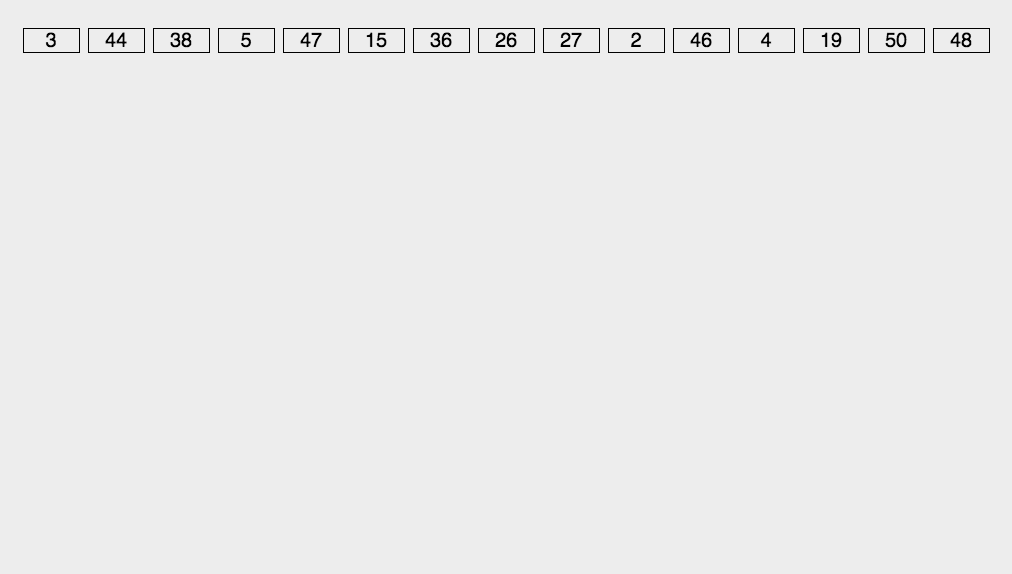10.3 代码实现

/**
* 基数排序
* @param array
* @return
*/
public static int[] RadixSort(int[] array) {
if (array == null || array.length < 2)
return array;
// 1.先算出最大数的位数；
int max = array;
for (int i = 1; i < array.length; i++) {
max = Math.max(max, array[i]);
}
int maxDigit = 0;
while (max != 0) {
max /= 10;
maxDigit++;
}
int mod = 10, div = 1;
ArrayList<ArrayList<Integer>> bucketList = new ArrayList<ArrayList<Integer>>();
for (int i = 0; i < 10; i++)
for (int i = 0; i < maxDigit; i++, mod *= 10, div *= 10) {
for (int j = 0; j < array.length; j++) {
int num = (array[j] % mod) / div;
}
int index = 0;
for (int j = 0; j < bucketList.size(); j++) {
for (int k = 0; k < bucketList.get(j).size(); k++)
array[index++] = bucketList.get(j).get(k);
bucketList.get(j).clear();
}
}
return array;
}


10.4 算法分析

MSD 从高位开始进行排序 LSD 从低位开始进行排序

• 基数排序：根据键值的每位数字来分配桶

• 计数排序：每个桶只存储单一键值

• 桶排序：每个桶存储一定范围的数值